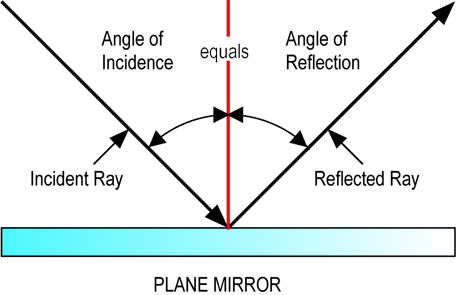# Refraction, reflection, and what is total reflection?

So if light travels from one media to another with a different refraction index, what may happen happen? Refraction, reflection or total reflection? I am quite confused as to the differences between these three concepts. Does 1 of these always happen in this situation? What exactly is total reflection and how/why does it occur?

• please no wikipedia links, too confusing! – Johny Kilkocx Sep 19 '12 at 6:09

Leaving aside total internal reflection for now, whenever light crosses a refractive index boundary there is always some reflection and some refraction.

Refraction is easily understood. In an earlier question you established that for any refractive index > 1 the speed of light in the medium $v$ is slower than $c$. However the frequency $f$ of the light remains the same. Since the wavelength $\lambda$ of the light is given by $\lambda = v/f$ this means the wavelength of the light decreases.As this diagram (shamelessly cribbed by Googling) shows, if the wavelength decreases the direction of the light ray has to change. The change of direction is called refraction.

For reflection, I can't think of a simple diagrammatic way to show why there is always reflection. When we're calculating the reflection we use the principle that the electric field of the light must be continuous at the boundary. If you add up the field on the two sides of the bounday you find that whenever there is refraction there is always some reflection to balance it out.

Total internal reflection only occurs when light is passing from a higher refractive index medium to a lower refractive index medium. The easiest way to see why it happens is described below.In the diagram above Snell's law tells us that:

$$\sin\theta_{air} = \frac{n_{water}}{n_{air}} \space \sin\theta_{water}$$

As light passes from the water into the air the light ray is always bent away from the normal and towards the surface of the water. The problem is that there is a value of $\theta_{water}$ at which the light is bent right round until it's parallel with the surface of the water i.e. $\sin\theta_{air} = 1$. This happens when:

$$\frac{n_{water}}{n_{air}} \space \sin\theta_{water} > 1$$

For values of $\theta_{water}$ greater than this critical value no light can escape from the water so all the light is reflected back into the water. This is the total internal reflection.

• Appreciate it, makes a lot more sense now. Thank you! – Johny Kilkocx Sep 19 '12 at 8:12

1) Reflection: When a beam of light strikes the surface of a transparent medium like glass or water which has a large number of closely spaced atoms, some of the light photons get scattered in backward direction from the interface (which separates air medium from water) which we guys call it as Reflection. Of course, most of the objects reflect visible light. The portion of reflected light waves vary accordingly to different interfaces. We could conclude that, "Without Reflection, we would be able to see nothing".

According to the laws of laws of reflection:

• The angle of incidence equals angle of reflection ($\theta_i=\theta_r$ or simply $i=r$). The incident ray, reflected ray and normal drawn to the surface at the point of incidence - all lie in the same plane.2) Refraction: Waves often travel from one medium to another. When they change medium, they often change directions. Light travels slowly in glass and other transparent objects than in air. This change of direction and their slow-motion is determined by the refractive index $\mu$ of materials.

$$\mu=\frac{C_a}{C_m}=\frac{\lambda_a}{\lambda_m}$$

For air, $\mu=1$ and for any other material other than air, $\mu>1$. It shouldn't be less than one. If it was, It'd have strongly disproved the Theory of Relativity. It should also be noted that the ray of light is deflected towards the normal when it passes from rarer medium to denser medium and viceversa. When you see a glass tank (filled with water) from the top, the bottom surface of the tank appears closer than it appears from either sides. Then, you should think of refraction..!

According to the laws of refraction:

• The incident ray, reflected ray and normal drawn to the surface at the point of incidence - all lie in the same plane. By Snell's law, ($i$ = angle of incidence, $r$ = angle of refraction) $$\mu=\frac{sin\theta_i}{sin\theta_r}$$

Let AB be the ray of light incident at an angle $i$ at a refracting medium XY of refractive index $\mu$ and refracted at an angle $r$. NN' be the normal drawn to the surface.(Rarer and Denser medium: Denser medium has the refractive index greater than that of the rarer medium. Denser medium should be any media other than air or vacuum)

3) Total Internal Reflection: When a ray of light AO passes from denser medium to rarer medium, light is partly reflected along OB and is refracted at $r$ along OC. As you can see, there are three cases... When the angle of incidence $i$ is increased, $r$ is also increased (As it passes from denser to rarer medium, light is deflected away from the normal NN'). As $i$ is further increased to some higher angle, $r$ becomes $90^o$ and the light ray grazes over the interface which separates the two media. This angle is called the critical angle (say $c$ or $\theta_c$). As the incident angle is increased above critical angle (i.e) $i>c$, $$r>90^o\implies sin\theta_r>1$$ which is impossible or no refraction is possible. Thus, the ray is reflected into the denser medium itself. This is called Total internal reflection.

According to wave theories, for Total internal reflection to happen:

• Light must travel from denser medium to rarer medium.
• Angle of incidence should be greater than critical angle (i.e) $i>c$.